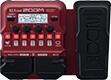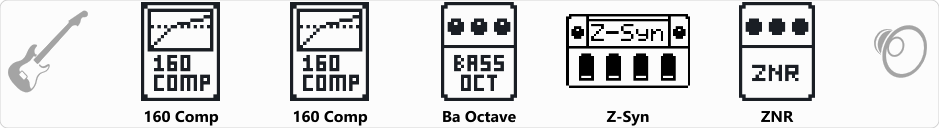# Chameleon Herbie Bass

Discussion in 'Zoom B1/B1X Four' started by julesbelan, Feb 9, 2023.

1. Chameleon Herbie BassDevice: Zoom B1X Four
Firmware: 2.00

Name on device: Chameleon
Optimized for: Guitar Amp

Effects chain:Chameleon Herbie Bass - Play with tone at 0 and humbucker if possible - play with RESO for more "wet" effect

Effect: "160 Comp" (Dynamics), active - "yes"
"Threshold" = -20
"Ratio" = 3.7
"Knee" = SOFT
"Volume" = 81

Effect: "160 Comp" (Dynamics), active - "yes"
"Threshold" = -22
"Ratio" = 1.5
"Knee" = HARD
"Volume" = 83

Effect: "Ba Octave" (Modulation), active - "yes"
"Oct" = 80
"Lo" = 0
"Hi" = 5
"Dry" = 30

Effect: "Z-Syn" (Sfx), active - "yes"
"FREQ" = 1
"Range" = 3
"Decay" = 14
"RESO" = 16
"Wave" = 0
"Tone" = 7
"BAL" = 75
"VOL" = 55
"Frequency" = 0
"Range" = 0
"Decay" = 0
"Resonance" = 0
"Wave" = SAW
"Tone" = 0
"Balance" = 0
"Volume" = 0

Effect: "ZNR" (Dynamics), active - "yes"
"Detect" = EFXIN
"Depth" = 100
"Threshold" = 30
"Decay" = 0

Patch Volume: 70